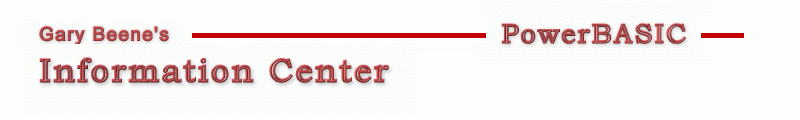## Remove Quotes

Category: Source Code Analysis

Date: 03-28-2012

'If you want to remove the quotes from source code and there are too many lines
'of code to do it manually, the following function will do the task for you.

'Primary Code:
'This function finds the first single-quote that is not embedded in double-quotes,
'and removes all of the text from that point forward. Pass it a string and it returns
'a string with the quote removed.
Function TrimQuotes(txt As StringAs String
Local i, qFlag As Long, c As String
For i = 1 To Len(txt)
c = Mid\$(txt,i,1)
If c = \$Dq AND qFlag = 1 Then
qFlag = 0
ElseIf c = \$Dq Then
qFlag = 1
ElseIf c = "'AND qFlag = 0 Then
Function = RTrim\$(Left\$(txt,i-1))   'found single-quote character not embedded in double-quotes
Exit Function
End If
Next i
Function = txt
End Function

'Compilable Example:
#Compiler PBWin 9, PBWin 10
#Compile EXE
#Dim All
#Include "Win32API.inc"

Global hDlg As DWord
Global iSelectLevel&, iDoLevel&, iForLevel&, iIfLevel&, iCaseLevel&, iWhileLevel&
Global iInterfaceLevel&, iMethodLevel&, iPropertyLevel&

Function PBMain() As Long
Local style&
style& = %WS_TabStop Or %WS_Border Or  %ES_Left Or %ES_AutoHScroll Or %ES_MultiLine Or %ES_NoHideSel Or %ES_WantReturn Or %WS_VScroll Or %ES_AutoVScroll
Dialog New Pixels, 0, "Test Code",300,200,300,300, %WS_OverlappedWindow To hDlg
Control Add TextBox, hDlg, 100, SampleText, 10,10,280,240, Style&
Control Add Button, hDlg, 200,"Trim", 80,260,100,20
Dialog Show Modal hDlg Call DlgProc
End Function

CallBack Function DlgProc() As Long
If CB.Msg = %WM_Command AND CB.Ctl = 200 AND CB.Ctlmsg = %BN_Clicked Then
Local temp\$
Control Get Text hDlg, 100 To temp\$
Control Set Text hDlg, 100, RemoveQuotes(temp\$)
End If
End Function

Function RemoveQuotes(txt As StringAs String
Local i As Long
Dim D() As String
i = ParseCount(txt, \$CrLf)
ReDim D(i-1)
Parse txt, D(), \$CrLf
For i = 0 To UBound(D)
D(i) = TrimQuotes(D(i))    'TrimQuotes() works on 1 line
Next i
Function = Join\$(D(), \$CrLf)
End Function

Function TrimQuotes(txt As StringAs String
Local i, qFlag As Long, c As String
For i = 1 To Len(txt)
c = Mid\$(txt,i,1)
If c = \$Dq AND qFlag = 1 Then
qFlag = 0
ElseIf c = \$Dq Then
qFlag = 1
ElseIf c = "'AND qFlag = 0 Then
Function = RTrim\$(Left\$(txt,i-1))   'found single-quote character not embedded in double-quotes
Exit Function
End If
Next i
Function = txt
End Function

Function SampleText() As String
Local temp\$
temp\$ = temp\$ + "Function MyF()" + \$CrLf
temp\$ = temp\$ + "If x = " + \$Dq + " ' " + \$Dq + " Then y=2 Else z=4  'comment" + \$CrLf
temp\$ = temp\$ + "While x=2 : y=3 : Wend  'comment" + \$CrLf
temp\$ = temp\$ + "Select Case x : ... : End Select  'comment" + \$CrLf
temp\$ = temp\$ + "For x=2 To " + \$Dq + "'5'" + \$Dq + " : Next i  'comment" + \$CrLf
temp\$ = temp\$ + "Do .. : " + \$Dq + " ' " + \$Dq + " : Loop While x=2  'comment" + \$CrLf
temp\$ = temp\$ + "Interface ... End Interface  'comment" + \$CrLf
temp\$ = temp\$ + "Method .." + \$Dq + " ' " + \$Dq + ". End Method  'comment" + \$CrLf
temp\$ = temp\$ + "Property ... End Property  'comment"
Function = temp\$
End Function

'gbs_00686
'Date: 03-10-2012

created by gbSnippets
http://www.garybeene.com/sw/gbsnippets.htm